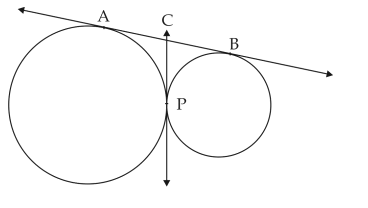# CBSE Class 10 Mathematics Sample Paper 2018 Set B

Read and download PDF of CBSE Class 10 Mathematics Sample Paper 2018 Set B designed as per the latest curriculum and examination pattern for Class 10 issued by CBSE, NCERT and KVS. The latest Class 10 Mathematics Sample Papers have been provided with solutions so that the students can solve these practice papers and then compare their answers. This will help them to identify mistakes and improvement areas in Mathematics Standard 10 which they need to study more to get better marks in Grade 10 exams. After solving these guess papers also refer to solved Class 10 Mathematics Question Papers available on our website to build strong understanding of the subject

## Mathematics Sample Paper Class 10 2018

Students can refer to the below Class 10 Mathematics Sample Paper designed to help students understand the pattern of questions that will be asked in Grade 10 exams. Please download CBSE Class 10 Mathematics Sample Paper 2018 Set B

### Mathematics Class 10 Sample Paper 2018

VSA:

1. If the common difference of an AP is 3, then what is  a15 - a9 ?

2. If the ratio between the length of the shadow of a tower and its height is √3 :1, then what is the angle of elevation of Sun?

3. The circumference of the base of a cone is 44 cm and the slant height is 25 cm. Find the curved surface area of the cone.

4. Find the area of a triangle whose vertices are (3, 0), (7, 0) and (8, 4).

SA–I:

1. Find the roots of the quadratic equation  3x2 -2√ 6x +2 = 0 .

2. Find the 4th term from the end of the AP -11, -8, -5, .........., 49.

3. In fig. 1, if length of AB is 9 cm, then, find the length of CP.4. A coin is tossed two times. Find the probability of getting atmost one head.

SA–II:

1 From a balloon vertically above a straight road, the angles of depression of two cars on the same side at an instant are found to be 45° and 60°. If the cars are 100 m apart, find the height of the balloon.

2. Find the area of the minor segment of a circle of radius 14 cm, when the angle of the corresponding sector is 60°.

3. A circular pond is 21 m in diameter. It is surrounded by 3.5 m wide path. Find the cost of constructing the path at the rate of Rs. 25 per m2.

4. A solid cylinder is of height 15 cm and diameter 7 cm. Two equal conical holes of radius 3 cm and height 4 cm are cut off, one from each circular end. Find the surface area of the remaining solid.

5. The surface area of a sphere of radius 5 cm is 5 times the curved surface area of a cone of radius 4 cm. Find the volume of the cone.

LA: 4

1. Anil takes 6 days less than the time taken by Varun to finish a piece of work. If both Anil and Varun together can finish that work in 4 days, find the time taken by Varun to finish the work independently.

2. Yasmeen saves Rs. 32 during the first month, Rs. 36 in the second month and Rs.40 in the third month. If she continues to save in this manner, in how many months she will save Rs. 2000, which she has intended to give for the college fee of her maid's daughter. What value is reflected here.

3. A train, travelling at a uniform speed for 360 km would have taken 48 minutes less to travel the same distance, if its speed were 5 km/h more. Find the original speed of the train.

4. Prove that the lengths of tangents drawn from an external point to a circle are equa